This is my second post on Word2Vec. The previous article was about the probabilistic model explaining the mechanics of embedding and appropriately using vector representation. You can find it here. In this part, we will review and implement skig -gram and negative sampling (SGNS) which is a more efficient algorithm for finding word vectors.

Introduction

SGNS is one of the most popular approaches for word embedding. Dense and distributed representation of words as vectors leads to many applications in NLP. Mikolov and the team of researchers developed the technique in 2013 at Google. SGNS is a refined model that improves the quality of representation and speed of computation. The main difference from earlier CBOW (Continuous Bag of Words) model is that the skip-gram model is designed to predict the context given a word where as in CBOW learns to predict the word by the context. This work was published in the paper ‘Distributed Representations of Words and Phrases and their Compositionality’.

Implementation

As in the first part consider a text consisting of characters from ‘a’ to ‘z’ sequentially [a, b,c, d, e, f, g, h, i, j, k,l, m, n, o, p, q, r,s, t, u, v, w, x, y, z], further let integers 0 to 25 represent the respective letters. Implementing skip-gram keeping the window size of 2, we can see that ‘a’ is related to ‘b’ and ‘c’, ‘b’ to ‘a’, ‘c’ and’ ‘d’ and so forth. It follows that one hot vector representing input words will be of dimension [26, 1]. With the help of the model, we will find the dense and distributed vector of size [10, 1]. The size of the embedding vector is selected arbitrarily.

The diagram below illustrates the context and target words.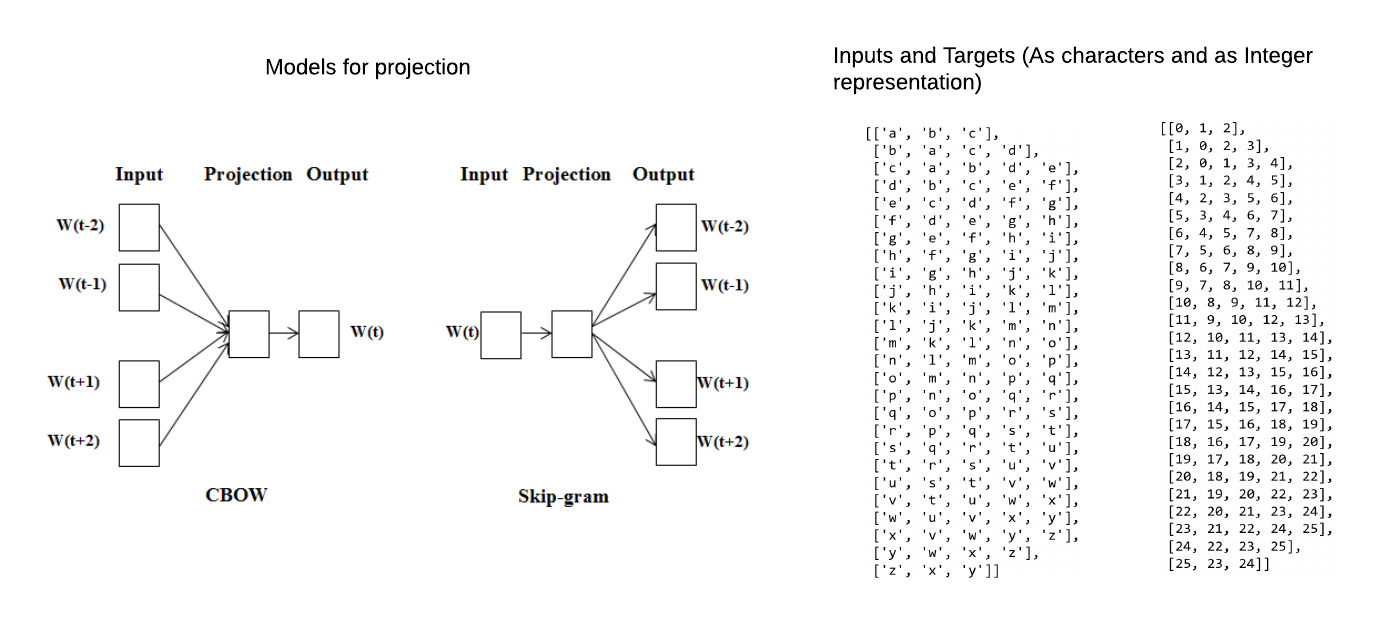Left. Arrangement of CBOW and Skip-gram models. Right. Character and corresponding integers grouped into lists of input and targets. For each, the first column represents the input character and the remaining items in each sub-lists are targets for the input.

It is easy to visualize the pattern of relationship. When they occur together within a span of 2 characters they are related or co-occurrence is true. The pattern is depicted in table and map below.Left. Characters occurring together are labeled 1 (not fully visualized). Right. The black color region implies distant (or no co-occurrence) and white region implies characters occurring together within the window of size 2 #Also note the diagonal symmetry — inputs and targets have symmetrical relation in this example.

The algorithm

The key feature of negative sampling is 2 embedding weight matrices. The first fully connected layer (FC1 -below) transforms input words to the embedding vector and the second weight matrix (FC2) transforms the target words to context vectors. The vector product of a pair of vectors representing input and target words is a score which passes through sigmoid activation. Model learns by comparing the final output with the target labels.

Backpropagation and update optimize the parameters/weights in both embedding matrices FC1 and FC2. Once fully trained -the whole model learns to predict the possible target words. However, the real purpose of training is to extract the embedding that is learned by the model and these vectors represent the dense and distributed representation of each word. Finally, the embedding vectors are used to find the similarities and other applications in NLP. First weight matrix(FC1) embed words as input and FC2 embeds words as targets.

Unlike the probabilistic model of Word2Vec where for each input word probability is computed from all the target words in the vocabulary, here for each input word has only few target words (few true and rest randomly selected false targets). The burden of computation is much lower and the model learns the embedding vector by contrasting true class with few random false targets. Also, random targets are not exhaustive and the model might never see all the negative targets. The key difference compared to the probabilistic model is the use of sigmoid activation as final discriminator replacing softmax function in the probabilistic model. (Refer to Word2Vec made easy for details of the probabilistic model)

The model is illustrated below.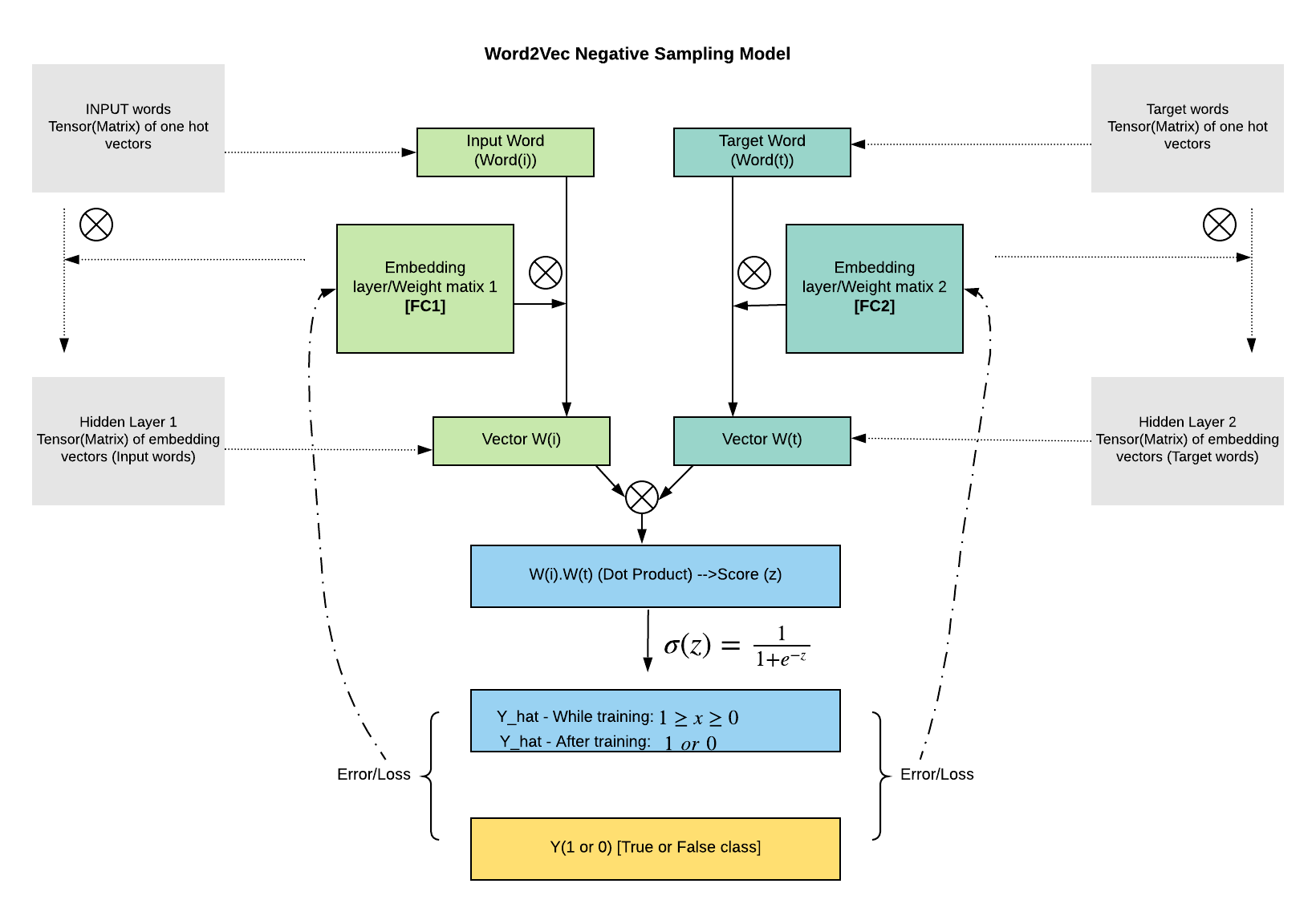The inner colored tiles represent information flow vector-wise or one word at a time, the outer grey tiles represent batches of inputs and targets and respective hidden layers as it occurs in loading and computing while implementing the algorithm in pytorch.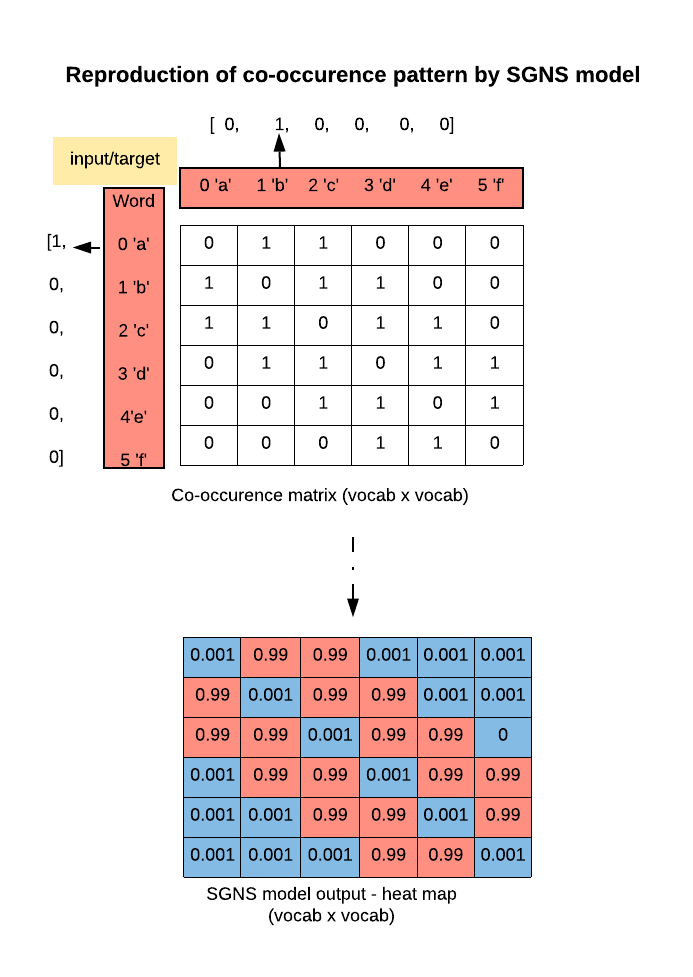Final output matrix closely resembles the original co-occurrence matrix, values are pushed close to 1 and 0s.

In code

#Generating input and true target sub-lists

`#Dependenciesimport numpy as npimport torchimport torch.nn as nnimport matplotlib.pyplot as plt%matplotlib inline`
`#Characters from 'a' to 'z' is represented by integers 0 to 25#Pairing input words with true targets and labeling this pair as 1`
`true_list = []`
`for i in range(26):`
`#Get the target indices given each input index    temp = []      a = i- 2    b = i - 1    c = i + 1    d = i + 2    temp.extend([a, b, c, d])        #Sub-lists of input and target and 1 as label    for j in range(4):        if temp[j] >=0 and temp[j] <=25:            true_list.append([i, temp[j], 1])`
`#print(true_list[:5])#[[0, 1, 1], [0, 2, 1], [1, 0, 1], [1, 2, 1], [1, 3, 1]]`

#Generating random(false) pairs and labeling them 0

`import random`
`#There are 98 true pairs, keeping size 400 selects more than 300 #random pairs or roughly 3 random targets for each input`
`def gen_rand_list(size = 400):`
`#true targets are filtered here as size of vocab is too small    false_list = []     for i in range(size):        frs = random.sample(range(26),1 )        sec = random.sample(range(26),1 )        if abs(frs - sec) > 2 or (frs == sec):            false_list.append([frs, sec, 0])    return false_list`

#Concatenating both lists (True and False pairs) followed by getting one-hot vectors of both input and targets

`def one_hot_auto():`
`#Joining and shuffling true and random input/target pairs`
`joint_list = np.concatenate((np.array(true_list), np.array(gen_rand_list())), axis = 0)    np.random.shuffle(joint_list)    inp_targ_labels = torch.Tensor(joint_list).long()`
`#Converting both inputs and targets to one-hot forms     #Two tensors are initialized as 0 tensors    #The item in i_th row whose index is equal to corresponding    #input/target is then replaced by 1`
`middle_word_arr = torch.zeros(inp_targ_labels.shape, 26)    sur_word_arr = torch.zeros(inp_targ_labels.shape, 26)    for i in range(len(inp_targ_labels)):        middle_word_arr[i, inp_targ_labels[i, 0]] = 1        sur_word_arr[i, inp_targ_labels[i, 1]] = 1    labels = inp_targ_labels[:, 2].float()    return (middle_word_arr, sur_word_arr, labels)`

#Defining network

`import torch.optim as optim#Create Network #2 fully connected layers with NO bias#Embedding dimension is 10#Rows of each weight matrix represents the embedding#Sigmoid is implemented using loss criterion (nn.BCELoss())`
`fc_inp_word = nn.Linear(26, 10, bias = False)fc_targ_word = nn.Linear(26, 10, bias = False)`
`LR = 0.001criterion = nn.BCELoss()params = list(fc_inp_word.parameters()) + list(fc_targ_word.parameters())optimizer = optim.Adam(params, lr = LR)`

#Train

`epochs = 10000print_every = 1000`
`#In skip-gram middle word becomes the input which predicts #surrounding words(targets)#Every time one_hot_auto() is called fresh batch is generated`
`mid_hot, sur_hot, labels = one_hot_auto()for i in range(epochs):`
`#Forward prop to get hidden layer    z_midl = fc_inp_word(torch.Tensor(mid_hot))    z_sur = fc_targ_word(torch.Tensor(sur_hot))        #Initialize a 1d matrix of 0s to store dot products between each    #row of first hidden matrix embedding input with second hidden    #matrix embedding target words    #This score forms the basis for optimization`
`dot_u_v = torch.zeros(mid_hot.shape, 1)    for j in range(len(z_midl)):        dot_u_v[j, :] = z_midl[j, :] @ z_sur[j, :]        #Sigmoid activation applied to dot products of vectors    desired_logits = dot_u_v    sig_logits = nn.Sigmoid()(desired_logits)        #Back prop and stepping    optimizer.zero_grad()    loss = criterion(sig_logits, torch.Tensor(labels).view(sig_logits.shape, 1))`
`loss.backward()    optimizer.step()    if i % print_every == 0:        print(loss.data)        #Scheduled one_hot_auto() to generate fresh random pairs         mid_hot, sur_hot, labels = one_hot_auto()`
`#Prints lossestensor(0.6029) tensor(0.0674) tensor(0.0146) tensor(0.0060) tensor(0.0029) tensor(0.0022) tensor(0.0011) tensor(0.0008) tensor(0.0003) tensor(0.0003)`

Visualizing the outputs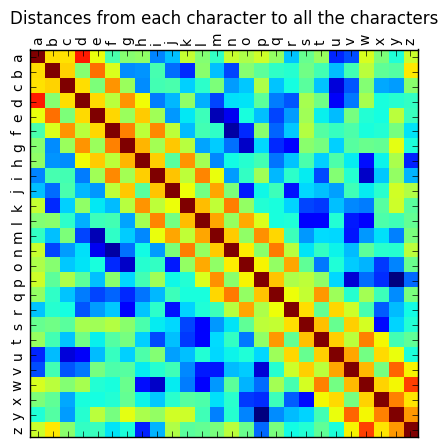Left. Score matrix a matrix of vector products -representing input words and target words (i.e. FC1 embedding and FC2 embedding). When the two vectors are neighbors the score is high (reddish) else low, clearly depicting the pattern of co-occurrence. Middle. Score matrix through sigmoid activation -all the high scores are pushed close to 1 and low scores close to 0. Note this matrix is a close copy of co-occurrence matrix we generated above from raw data. Right. This matrix depicts distance from each character to every character including itself. Unlike before here only FC1 (embedding input words) is used. The diagonal values are the product with itself (has the highest value of 1). The cells adjacent to diagonal are close neighbors and more similar while the rest of the elements have low or negative distances.

Real world example

For experimentation, I selected the book ‘world order’ by Henry Kissinger and got the word embedding using the exact algorithm as above. Here is the pseudo-code for processing the book and finding vectors.

`#Psuedo-code for implementation#Download the book in pdf#Convert it to csv(I find working with csv simpler)#import csv --> read the book#import re --> convert whole text to lower case, remove digits and punctuation#from collections import Counter --> Count word frequency and find most common words#stop_words = Most frequent words (for  example: top 20), add or remove more words as necessary#uniq_words --> From the corpus above find set of unique words#Indices of uniq_words as used as look up from words to integers#Build a word gram comprising input word and its true target and label it as 1#Build another list consisting of input words, random indices as false targets and label 0#Join the both lists and shuffle and feed in batches#fc1 = nn.Linear(len(uniq_words), embed_size, bias = False)--> represents input words#fc2 = nn.Linear(len(uniq_words), embed_size, bias = False)--> represents the target words or contexts#criterion, optimizer and network as described in the post#Finding distances --> find index of any word in uniq_words -->nn.CosineSimilarity(dim = 0)(fc1.weight.t()[idx, :], fc1.weight.t()[i, :])`

For quick implementation, only cpu was used and ran the network for a couple of hours. The window size was 5, meaning the target word for input are 5 words before and 5 words after the selected word. K or negative samples was just 2(2 random pairs were given for every true pair, and it was intentionally kept low to see if it is still able to extract the meaningful representation).

Find similar words

find_dist(‘nuclear’, 5): [‘nuclear’, ‘proliferation’, ‘age’, ‘challenge’, ‘khomeini’]

find_dist(‘mankind’, 5): [‘mankind’, ‘acting’, ‘perspective’, ‘longer’, ‘concept’]

find_dist(‘henry’, 5): [‘henry’, ‘kissinger’, ‘history’, ‘reflections’, ‘character’]

find_dist(‘khomeini’, 10): [‘khomeini’, ‘statecraft’, ‘iranian’, ‘tradition’, ‘approaches’, ‘iran’, ‘nuclear’, ‘vision’, ‘proliferation’, ‘revolution’]

The embedding outputs similar words that are quite consistent given the nature of the text.

Here is the github link to full code for implementation:https://github.com/LakheyM/word2vec/blob/master/word2vec_SGNS_git.ipynb

Further consideration

Adjusted sampling: here frequency of each word is adjusted by some power (generally 3/4). Drawing a random negative sample according to adjusted frequency instead of unigram distribution improves the quality of embedding probably due to increased probability for selecting less frequent words. To implement adjusted sampling — add or remove words from corpus as per the difference between two frequencies(unigram vs adjusted). Shuffle, index and finally take k random indices as negative samples. The equations are given below.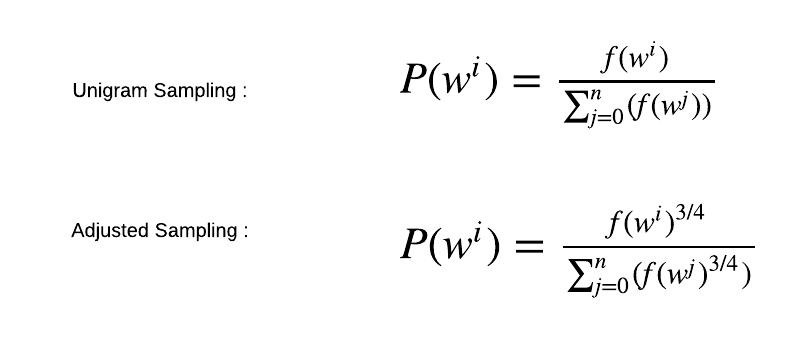Unigram and adjusted sampling. P(wi): Probability of selecting the i.th word, f(wi): frequency of word i, Summation from 0 to n represents a global count of words. As seen the in adjusted sampling the frequency is raised to the power of 3/4.

Hierarchical softmax: It is an alternative to negative sampling. Just like negative sampling it improves computational efficiency and the cost is only O(log(|V|)), which correspond to the depth of the path from the root to a leaf node. This binary tree representation of words in the vocabulary is the key feature of Hierarchical softmax.

Unique path links from root to leaf and each node is associated with a vector that is learned by the model. The vector product and sigmoid activation give balanced probabilities on either side of the node. Finally, likelihood is computed by multiplying all the probabilities in the path. In the picture below, the probability of a word w given a vector C, P(w|C), is equal to the probability of a random walk starting in the root and ending in the leaf node corresponding to w.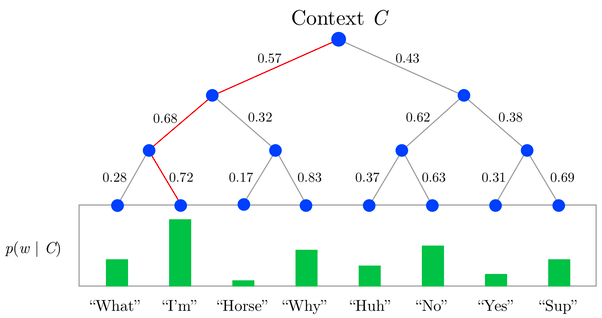To evaluate the probability of a given word, take the product of the probabilities of each edge on the path to that node: P(I’m|C) = 0.57 * 0.68 * 0.78 = 0.28. Note that paths are shared the nodes or branch at nodes such that either same probability or complementary probability is used saving lots of computational resources. (Source: https://www.quora.com/What-is-hierarchical-softmax)

Conclusion

Word2Vec and similar algorithms are the key steps in implementing all sorts of NLP tasks. With the use of negative sampling it is possible to get the representation of whole data relatively quickly and/or using less computing power. Perhaps the biggest advantage of implementing embedding from the scratch is that it gives immense flexibility and control while developing end to end NLP applications.

References

 Mikolov, Tomas, Ilya Sutskever, Kai Chen, Gregory S. Corrado and Jeffrey Dean. “Distributed Representations of Words and Phrases and their Compositionality.” NIPS(2013).

 CS224n: Natural Language Processing with Deep Learning, Winter 2017. ( https://tensorflowkorea.files.wordpress.com/2017/03/cs224n-2017winter-notes-all.pdf)

Also check out: Ali Ghodsi, Lec 13: Word2Vec Skip-Gram